# Kerneldensityestimate.jl

Kernel Density Estimate with product approximation using multiscale Gibbs sampling
Alternatives To Kerneldensityestimate.jl
Bcpd218
2 months ago9mitC
Bayesian Coherent Point Drift (BCPD/BCPD++/GBCPD/GBCPD++)
4 years agoPython
Code for performing 3 multitask machine learning methods: deep neural networks, Multitask Multi-kernel Learning (MTMKL), and a hierarchical Bayesian model (HBLR).
Approxbayes.jl44
a year ago12otherJulia
Approximate Bayesian Computation (ABC) algorithms for likelihood free inference in julia
Simpleabc29
7 years agomitJupyter Notebook
A Python package for Approximate Bayesian Computation
Bkmr23
a year ago7R
Bayesian kernel machine regression
Kerneldensityestimate.jl22
9 months ago10lgpl-2.1Julia
Kernel Density Estimate with product approximation using multiscale Gibbs sampling
Gpsig14
3 years ago1apache-2.0Jupyter Notebook
Bayesian Learning from Sequential Data using Gaussian Processes with Signature Covariances
Bmtmkl13
5 years agoR
Bayesian Ntk13
2 years agoJupyter Notebook
Code to accompany paper 'Bayesian Deep Ensembles via the Neural Tangent Kernel'
Herding Paper11
7 years agomitTeX
Alternatives To Kerneldensityestimate.jl
Select To Compare

Alternative Project Comparisons

# KernelDensityEstimate.jl

Kernel Density Estimation with product approximation using multiscale Gibbs sampling.

All code is implemented in native Julia, including plotting. The main focus of this module is the ability to take the product between multiple KDEs, and makes this module unique from other KDE implementations. This package also supports n-dimensional KDEs. Please see examples below for details. The implementation is already fairly optimized from a symbolic standpoint and is based on work by:

``````Sudderth, Erik B.; Ihler, Alexander, et al. "Nonparametric belief propagation." Communications of the ACM 53.10 (2010): 95-103.
``````

## Installation

In Julia 1.0 and above:

``````] add KernelDensityEstimate
``````

## Plotting Functions

The plotting functions for this library have been separated into KernelDensityEstimatePlotting.jl. Plotting functionality uses Gadfly. Comments welcome.

# Examples

Bring the module into the workspace

``````using KernelDensityEstimate
# Basic one dimensional examples
# using leave-one-out likelihood cross validation for bandwidth estimation
p100 = kde!([randn(50);10.0.+2*randn(50)])
p2 = kde!([0.0;10.0],[1.0]) # multibandwidth still to be added
p75 = resample(p2,75)

# bring in the plotting functions
using KernelDensityEstimatePlotting
plot([p100;p2;p75],c=["red";"green";"blue"]) # using Gadfly under the hood
``````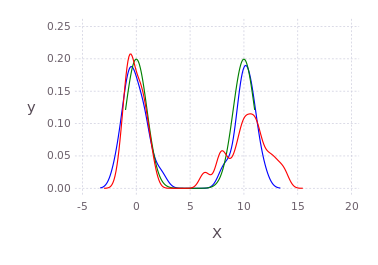Multidimensional example

``````pd2 = kde!(randn(3,100));
@time pd2 = kde!(randn(3,100)); # defaults to loocv
pm12 = marginal(pd2,[1;2]);
pm2 = marginal(pm12,);
plot(pm2);
``````

Multiscale Gibbs product approximation example

``````p = kde!(randn(2,100))
q = kde!(2.0.+randn(2,100))
dummy = kde!(rand(2,100),[1.0]);
mcmciters = 5
pGM, = prodAppxMSGibbsS(dummy, [p;q], nothing, nothing, Niter=mcmciters)
pq = kde!(pGM)
pq1 = marginal(pq,)
Pl1 = plot([marginal(p,);marginal(q,);marginal(pq,)],c=["red";"green";"black"])
``````

Direct histogram of points from the product

``````using Gadfly
draw(PDF("product.pdf",15cm,8cm),hstack(Pl1,Pl2))
``````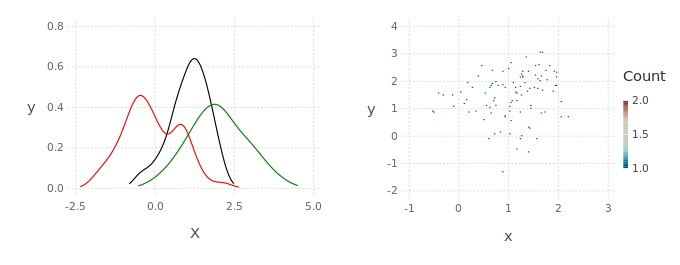KDE product between non-gaussian distributions

``````using Distributions
p = kde!(rand(Beta(1.0,0.45),300));
q = kde!(rand(Rayleigh(0.5),100).-0.5);
dummy = kde!(rand(1,100),[1.0]);
pGM, = prodAppxMSGibbsS(dummy, [p;q], nothing, nothing, Niter=5)
pq = kde!(pGM)
plot([p;q;pq],c=["red";"green";"black"])
``````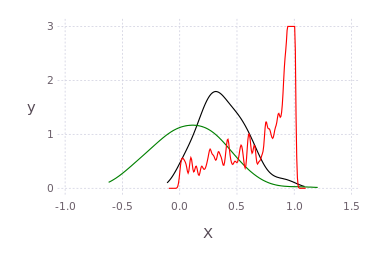Draw multidimensional distributions as marginalized 2D contour plots

``````axis=[[-5.0;5]';[-2.0;2.0]';[-10.0;10]';[-5.0;5]']
draw(PDF("test.pdf",30cm,20cm),
plot( kde!(randn(4,200)) ) )

N=200;
pts = [2*randn(1,N).+3;
[2*randn(1,round(Int,N/2))'.+3.0;2*randn(1,round(Int,N/2))'.-3.0]';
2*randn(2,N).+3];
p, q = kde!(randn(4,100)), kde!(pts);
draw(PNG("MultidimPlot.png",15cm,10cm),
plot( [p*q;p;q],c=["red";"black";"blue"], axis=axis, dims=2:4,dimLbls=["w";"x";"y";"z"], levels=4) )
``````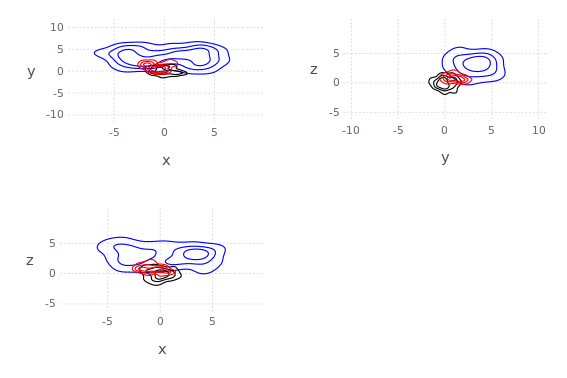``````# or draw product natively
draw(PNG("MultidimPlotProd.png",10cm,7cm),
plot( p*q, axis=axis, dims=[2;4],dimLbls=["w";"x";"y";"z"]) )
``````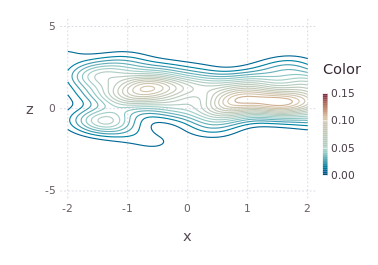# Contributors

The original C++ kde package was written by Alex Ihler and Mike Mandel in 2003, and has be rewritten in Julia and continuously modified by Dehann Fourie since.

Thank you to contributors and users alike, comments and improvements welcome according to JuliaLang and JuliaRobotics standards.

Popular Kernel Projects
Popular Bayesian Projects
Popular Operating Systems Categories
Related Searches

Get A Weekly Email With Trending Projects For These Categories
No Spam. Unsubscribe easily at any time.
Kernel
Julia
Bayesian
Plotting
Probabilistic Programming